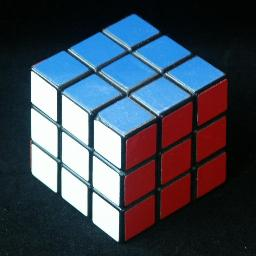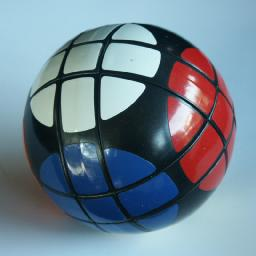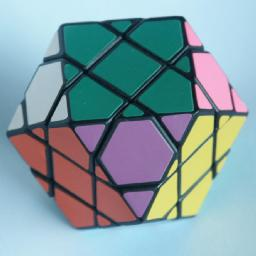# Rubik's Cube 3x3x3This is the puzzle that started it all. It was invented by Ernő Rubik in Hungary around 1974, the patent was filed 30 January 1975, HU 170,062 and eventually produced and marketed by Ideal Toys in the early 1980s. It was hugely successful, and many copycat cubes were made, such as the one pictured above. It is estimated that more than 100 million cubes were made, and it is still being manufactured today.

The Rubik's Cube is a cube which is built from smaller cubes, 3 to an edge, i.e. a 3x3x3 cube. The 9 pieces on each face can rotate, which rearranges the small cubes at that face. The six sides of the puzzle are coloured, so every corner piece shows three colours, every edge piece shows 2 colours, and every face centre only one.

Turning a face does not change the face centres (their twist is not a visible change of pattern) so these can be considered solved already, the other pieces have to be placed correctly around them.

## The number of positions:

There are 8 corner pieces with 3 orientations each, 12 edge pieces with 2 orientations each, giving a maximum of 8!·12!·38·212 positions. This limit is not reached because:
• The total twist of the cubes is fixed (3)
• The total number of edge flips is even (2)
• The pieces have an even permutation (2)

This leaves 8!·12!·37·210 = 43,252,003,274,489,856,000 or 4.3·1019 positions.

There are some 'Picture Cubes', Rubik's cubes which have pictures or some other design on the faces. A picture cube is more difficult to solve because the orientation of the face centres is visible. If these orientations are taken into account then there are 46/2 = 2048 times as many positions. The division by 2 is because of another parity restriction - the number of quarter turns of the centres must have the same parity as the permutation of the corners.

There are some variations of the puzzle that have the same mechanism but which differ in shape. The Rubik's World is also shown above, and is a ball-shaped picture cube, i.e. the centres have a visible orientation, and can be solved just like any normal picture cube. Two other examples are the ordinary ball-shaped 'cube', and the diamond puzzle (a cuboctahedron), both of which are shown above. These two can be solved in the exact same way as the normal Rubik's cube. Some variations, for example the Barrel/Octagon, has some pieces of which the orientation is not discernible, which may cause a bit of difficulty when first encountered. Other variations such as the Diamond Style Puzzler have many identical pieces which makes it much easier.

If you browser supports Java, then you may wish to play with my Rubik's Cube applet by opening the link below:

## Cubie Java Applet

There is little I can add to the massive amount written about the cube, both in books and on the internet. For completeness I will nevertheless give two solutions here. They are by no means fast, but are typical examples of cube solutions.

## Notation:

I will use the standard notation for cube moves, as introduced by David Singmaster. Let the faces be denoted by the letters L, R, F, B, U and D (Left, Right Front, Back, Up and Down). Clockwise quarter turns of a face are denoted by the appropriate letter, anti-clockwise quarter turns by the letter with an apostrophe (i.e. L', R', F', B', U' or D'). Half turns are denoted by the letter followed by a 2 (i.e. L2, R2, F2, B2, U2 or D2).

## Solution 1:

In layers.
Phase 1: Solve the top layer edges.
This phase is straightforward, but I will nevertheless give a full description.
1. Find an edge piece that belongs on the top face but which is not already there. If there are none, but they are not all positioned correctly, then give the side containing one of the incorrect ones a half turn.
2. If that edge is in the bottom layer then rotate D to place the piece below its destination, and then hold the cube so that both the piece and its destination are in the front face.
1. To move DF to UF, do F2.
2. To move FD to UF, do U'F'RF.
If instead that edge is in the middle layer then hold the cube so that the piece is at the front right, and do one of the following:
1. To move RF to UF, do F'.
2. To move RF to UR, do UF'U'.
3. To move RF to UB, do U2F'U2.
4. To move RF to UL, do U'F'U.
5. To move FR to UR, do R.
6. To move FR to UB, do URU'.
7. To move FR to UL, do U2RU2.
8. To move FR to UF, do U'RU.

With experience you can save many turns of the U face and choose the order in which you solve the edges so that this phase usually takes no more than 7 or 8 moves in total.

Phase 2: Solve the top corners.

1. Find a corner piece in the bottom layer that belongs in the top layer. If there are none, and not all four are correct yet then hold the cube so that an incorrect corner is at UFR. If the colour of the face is at the front of the piece then do R'DR otherwise do R'D'R.
2. Rotate D to place the corner piece below its destination, and hold the cube so that the piece and its destination are at the front right. Then do one of the following:
1. To move FRD to URF, do FDF'.
2. To move RDF to URF, do R'D'R.
3. To move DFR to URF, do FD'F'R'D2R.

By solving corner pieces which don't show the U colour on the D face first, the longer b3 case is often avoided.

Phase 3: Solve the middle edges.

1. Find an edge piece in the bottom layer that belongs in the middle layer. If there are none, and the middle layer is not correct then choose any of the bottom edges to displace a wrong piece in the middle layer. Hold the cube so that the destination of the edge is at the front right, and then rotate D to bring the edge piece at the front.
2. Do one of the following to place the edge correctly.
1. To move FD to FR, do D'R'DR DFD'F'.
2. To move DF to FR, do D2FD'F' D'R'DR.

Phase 4: Position the bottom corners.

1. Rotate D until at least two corners are positioned correctly, ignoring their orientations.
2. If you need to swap two corners, then do one of the following:
1. To swap DLF and DFR, do R'D'R FDF' R'DR D2.
2. To swap DLF and DRB, do R'D'R FD2F' R'DR D.

Phase 5: Orient the bottom corners.

1. If there are four twisted corners then hold cube so that a corner which is clockwise twisted at the front left (the D colour shows on the left of that corner).
If there are three twisted corners then hold cube so that the corner which is not twisted at the front left (the D colour shows on the bottom of that corner).
If there are two twisted corners then hold cube so that the corner which is anti-clockwise twisted at the front left (the D colour shows on the front of that corner).
2. Perform R'D'RD'R'D2RD2.
3. Repeat a-b if necessary until the corners are solved.

Phase 6: Position the bottom edges.

1. Do one of the following:
1. To swap DL-DR and DF-DB, do L2R2U2L2R2 D L2R2U2L2R2 D'
2. To swap DF-DR and DL-DB, do R2L2 U F2R2L2B2R2L2 U' R2L2
3. To cycle DR->DB->DL->DR, do L'RFLR' D2 L'RFLR'.
4. To cycle DL->DB->DR->DL, do L'RF'LR' D2 L'RF'LR'.

You need not use sequences 1 and 2 since they can be solved by applying 3 or 4 twice.

Phase 7: Orient the bottom edges.

1. Do one of the following:
1. To flip DF, DR, do FU'DR2U2D2L D' L'D2U2R2D'UF' D.
2. To flip DF, DB, do FU'DR2U2D2L D2 L'D2U2R2D'UF' D2.
3. To flip all four edges, apply either of the above twice.

Phase 8: Orient the face centres.
This phase is only necessary on picture cubes, or Rubik's World, where the face centres have a visible orientation.

1. If two face centres need twisting, then hold the cube such that one of the centres is on the top and the other is either on the bottom or the right face. Then do one of the following:
1. To turn centres U and R', do RL'FB'UD' R' U'DF'BR'L U.
2. To turn centres U2 and R2, do RL'FB'UD' R2 U'DF'BR'L U2.
3. To turn centres U' and R, do RL'FB'UD' R U'DF'BR'L U'.
4. To turn centres U and D', do RL'F2B2RL' U RL'F2B2RL' D'.
5. To turn centres U2 and D2, do RL'F2B2RL' U2 RL'F2B2RL' D2.
6. To turn centres U' and D, do RL'F2B2RL' U' RL'F2B2RL' D.
Note that it is possible to take care of the top and side centres during the first 7 phases, so that at most only the bottom face centre needs twisting. This step is then unnecessary.
2. If any face centre needs a half turn, then hold the cube with that face at the top, and do the following:
To turn centre U2, do RLU2R'L'U RLU2R'L'U.

There are many similar solutions that solve the cube in layers. Most are a lot faster than this one. Speed can be improved in several ways:

• Use more sequences for orienting the final corners, and in general use shorter sequences for phases 4-7.
• Phase 2 and 3 can be combined, so that top corners and middle edges are first placed adjacent in the bottom layer and then slotted into position as a single unit. I generally use this method myself.
• Phase 2 and 3 can be combined in a different way: First solve 3 top corners. Then place the three adjacent middle edges correctly, which uses short move sequences because of the unsolved corner. Each edge is done by turning U to place the unsolved corner above the edge destination, and then using three (or four) moves to place the edge. Once these 3 edges are done, the final corner/edge pair is put together and solved as a unit.
• It is also possible to combine phase 1 with 2 and 3, by first building a 2×2×2 block, then a 2×2×3 block, and only then the last edge of the top layer. See Lars Petrus' web site.
• Phase 4-7 can be combined in several different ways, nearly all of which involve memorising a large number of sequences.
You could position all the bottom pieces correctly first (23 sequences), and then orient them (57 sequences). See the book 'Winning Ways Vol.2' by Berlekamp, Conway and Guy.
You could do this in the opposite order too, which is a bit better; orient them first (57 sequences) and then positioning them (23 sequences). This is better because it is easier to recognise the situation in this order. See for example Jessica Fridrich's web page.
You could solve the corners first (42 sequences) and then solve the edges (29 sequences).
You could do this in the opposite order too; edges first (21 sequences) and then corners (86 sequences), but there are rather many sequences needed to do the corners in one step. You could however position (4) and orient (7) the corners in two steps (in either order) while leaving the edges untouched.
• Another approach is that used by Lars Petrus on his web page. He solves the top two layers except for one corner/edge pair. He then uses the unsolved column to orient the edges. He then solves the column, and solves the final layer like phases 4-6, except that all the sequences he uses do not change the edge orientations. As far as I know his method is the only fast solution that does not involve a large amount of memorisation. I mostly use his method to solve the cube's final layer.

Below is a corners first algorithm, i.e. all the corners are solved first, then the edges. Conceptually this is a good idea, since it can use slice moves (moves of a middle layer) which only involves edges. This kind of solution is relatively easy to understand and remember. They can be quite fast because of this, but they do generally use more moves than layer methods because turns of a slice are often counted as two moves.

## Further notation:

The only slice moves in the following solution are moves of the middle layer, i.e. the layer between U and D. Let M denote a clockwise quarter turn of the middle layer when looking from above (the same direction as the move U).

## Solution 2: Corners then edges.

Phase 1: Solve the corners.
Use any method you like. Use part of solution 1, or use the method for the mini cube. Afterwards the top and bottom centres should match their corners, but the centres in the middle layer need not match.

Phase 2: Solve the edges in the U/D layers.

1. Find the edge piece that belongs at the front top. If it does not lie in the middle layer then rotate the whole cube around the U/D axis to bring it to the front face and do FM2F'.
2. Hold the cube with the destination of the piece at the front top and turn M to bring the piece to the back right. Then do one of the following:
1. To move BR to UF, do FMF'
2. To move RB to UF, do F'M2F.
3. Find the edge piece that belongs at the front bottom. If it already lies at the front bottom except upside down, then do F'M'F M' FM'F' to flip it around. If it does not lie in the middle layer and not at the bottom front then rotate the whole cube around the U/D axis to bring it to the front face and do FM2F'.
4. Hold the cube with the destination of the piece at the front bottom and turn M to bring the piece to the back right. Then do one of the following:
1. To move BR to DF, do F'M'F M F'M F
2. To move RB to DF, do F'M'F2 M'F'
5. Repeat a-d for each pair of edges in the U/D layers.

Phase 3: Solve the middle layer.

1. Turn M to place the centres correct with respect to the U/D layers.
2. Place the edges correct by doing one of the following:
1. To swap FR-BL, FL-BR do M L2R2 M' L2R2.
2. To swap FR-BR, FL-BL do R2 M2 R2 M2.
3. To cycle FL->FR->BR->FL do R2 M' R2 M.
4. To cycle FR->FL->BR->FR do M' R2 M R2.
3. Orient the edges by doing one of the following:
1. To flip FR and BR do RM'RM'RM'RM2 RM'RM'RM'R.
2. To flip FL and BR do RM'RM'RM'RM RM'RM'RM'RM.
3. To flip FR, FL, BR, BL do BUB' RM'RM'RM'RM' BU'B'.

There are not as many variations of the corners first algorithm as there were with the layers algorithm, but the speed of this algorithm can be improved in several ways.

• Phase 2 can be speeded up simply by placing the edge belonging at UF in the FD position first, and when you next place the correct piece at FD, the first piece will be displaced and end up correctly at UF.
• Another variation of phase 2 involves solving the edges singly instead of in pairs. Its basic idea is to rotate the layer opposite the destination to bring an unsolved edge to the front. This way you can solve 3 edges in each layer singly without disturbing solved edges. The final two edges are then placed as before.
• Phase 3 can be done quicker by using shorter sequences, or by memorising sequences that place and flip edges at the same time. There are only 20 sequences needed for this.